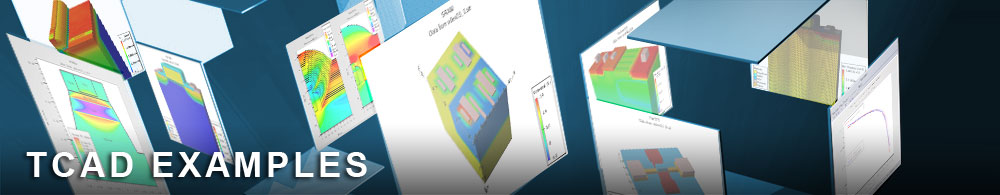VCSEL using Gain Guiding

## vcselex03.in : VCSEL using Gain Guiding

Requires: Blaze / VCSEL
Minimum Versions: Atlas 5.28.1.R

This example demonstrates the basic DC and optical characteristics of a Vertical Cavity Surface Emitting Laser (VCSEL) and simulates the self heating effects due to the power disipated by the diode. It demonstrates:

• VCSEL structure definition using Atlas syntax
• Material parameter specification
• Choosing the optical and electrical physical models
• Initial biasing of the device
• Activating the VCSEL module of Atlas
• Activation of the gain guiding model using the LASER statement
• Calculation of laser electrical and optical characteristics
• Calculation of self heating effects from the power dissipated in the diode

The first half of the input file describes the Atlas structual syntax, including the mesh, region electrode and doping commands that are also common to other Atlas examples. LASER specific structual commands include the powerful DBR statements which allow the definition of the numerous periodic semiconductor hetero-structure layers that make up the two mirrors of a VCSEL and it's active region respectively, each in a single line of the input file. VCSELs are modelled using a pseudo-3D method which assumes the device has cylindrical geometry and symetry. This is activated using the MESH CYL statement. The point X=0 represents the center of the device. The mesh statements define the mesh in the X direction only. Mesh in the Y direction is defined using SY=<n> parameter the REGION statements. This creates "n" number of mesh points in the Y direction in the region to which it refers. DBR statements also contain Y mesh parameters and are described below.

The REGION statements contain "TOP" or "BOTTOM" parameters to define the location of the region. The device can therefore have the new region being defined attached to either the top or bottom of the device. Donor or acceptor doping can also be assigned in each region statement using the DONOR=<n> or ACCEPT=<n> parameters respectively.

The Distributed Bragg Reflectors (DBRs) are defined using the DBR statement. The DBR statement allows the definition of a mirrors consisting of alternating layers of two materials with differing refractive index. The number of layers is defined by the HALF.CYC=<n> parameter. The thickness of each type of layer is defined by the THICK1=<n> and THICK2=<n> parameters respectively. The number of mesh points in the Y direction are defined by the N1=<n> and N2=<n> parameters respectively. The materials of each layer are defined by the MAT1=<material> and MAT2=<material> parameters. Mole fractions for ternary and quaternary materials are defined by X1.COMP=<n>, X2.COMP=<n> Y1.COMP=<n> and Y2.COMP=<n> parameters. Donor or acceptor doping is defined by ND1=<n>, ND2=<n>, NA1=<n> and NA2=<n> parameters respectively.

The active region of the VCSEL consists of one or more quantum wells. In this example, the active region contains 6 wells that are defined by the QWELL parameter on the associated REGION statements. The sampling for Schrodinger solutions is given by the WELL.NX and WELL.NY parameters of the REGION statement. The gain model for the active quantum wells is specified by the LI parameter on the MODEL statement. The SPONT parameter specifies that the gain model will be used to model spontaneous recombination.

In order to maximize their reflectivity, the DBRs must be defined such that intensity peaks and troughs of the standing wave light pattern fall at the boundary between the two dissimilar semiconductors. The standing wave light pattern for each mode is a function of the light propogation velocity and the thickness of each layer of the DBR. The ratio of light propogation velocity in a vacuum compared to each layer in the DBR is given by the refractive index, which in turn is the square root of the permittivity of the material at the wavelengths concerned. The permittivity of each semiconductor is defined by the ESPINF=<n> parameter in the MATERIAL statement. It should be noted that this is the optical permittivity which can be significantly lower than the permittivity at electrical frequencies. The thermal properties of the materials, necessary for calculating local temperature rises, are also defined on the material statement in common with other thermal simulations.

Heat loss from the device is defined using thermal contacts in the THERMCONTACT statement in the usual way, which specifies thermal conductivities to simulate heat loss from radiation via exposed surfaces or conduction through the semiconductor to a heatsink.

Once the structure has been defined, a number of parameters regarding the photonic equations are defined in the VCSEL statement. The photonic energy range over which the user wishes to look for standing wave patterns is defined by the EINIT=<n> and EFINAL=<n> parameters. There is little point in investigating photonic energies less than the bandgap of the active region, as these will not lase.

During execution the program stores structure files at various biases so that the user can track which standing wave pattern has the most gain both before and after lasing occurs. The standing wave pattern associated with lasing in the fundamental mode usually consists of an envelope function with a single large peak of light output located at the active region. Lasing is also associated with a sudden constancy of the optical gain with bias which can be plotted in TonyPlot using the creatd LOG FILE. Typical parameters that can be viewd in TonyPlot using the created structure and log files are:

• DC I-V diode characteristics
• Light power versus electrical bias
• Optical gain versus electrical bias
• Light intensity distributed over the structure
• Local device temperature in structure files or
• Maximum device temperature versus electrical bias in log files

To load and run this example, select the Load button in DeckBuild > Examples. This will copy the input file and any support files to your current working directory. Select the Run button in DeckBuild to execute the example.